Students can download Maths Chapter 5 Statistics Ex 5.3 Questions and Answers, Notes, Samacheer Kalvi 6th Maths Guide Pdf helps you to revise the complete Tamilnadu State Board New Syllabus, helps students complete homework assignments and to score high marks in board exams.

## Tamilnadu Samacheer Kalvi 6th Maths Solutions Term 1 Chapter 5 Statistics Ex 5.3

Question 1.
Read the given Bar Graph which shows the percentage of marks obtained by Brinda in different subjects in an assessment test.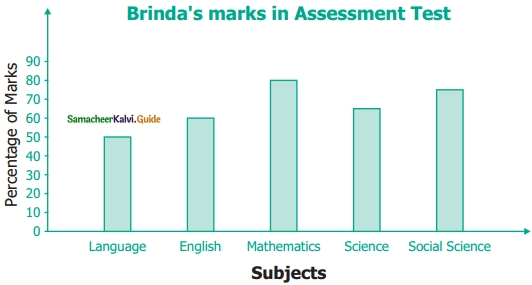Observe the Bar Graph and answer the following questions.
(i) 1 Unit = ………… % of marks on vertical line.
(ii) Brinda has scored maximum marks in …….. subject.
(iii) Brinda has scored minimum marks in …….. subject.
(iv) The percentage of marks scored by Brinda in Science is ……..
(v) Brinda scored 60 % marks in the subject ……..
(vi) Brinda scored 20% more in …….. subject than ……… subject.
Solution:
(i) 10
(ii) Mathematics
(iii) Language
(iv) 65%
(v) English
(vi) Mathematics, ScienceQuestion 2.
Chitra has to buv Laddus in order to distribute to her friends as follows: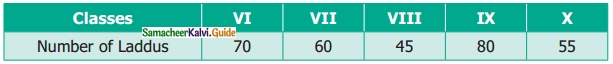Draw a Bar Graph for this data.
Solution:
Distribution of Laddus by Chitra to her friends
Scale : 1 unit = 10 Laddus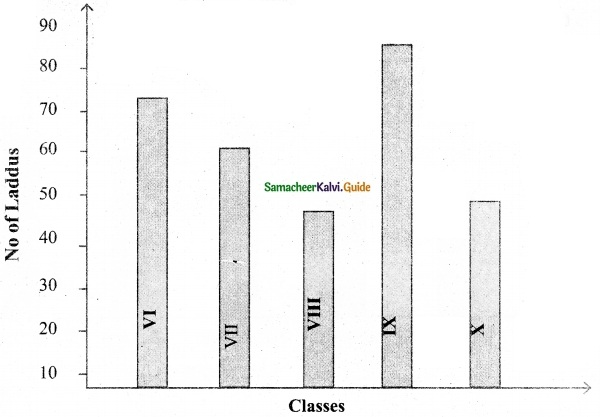Question 3.
The fruits liked by the students of a class are as follows: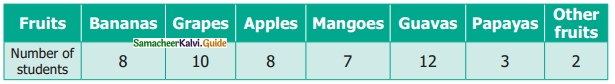Solution:
Draw a bar graph for data this data
Scale 1 unit = 2 fruits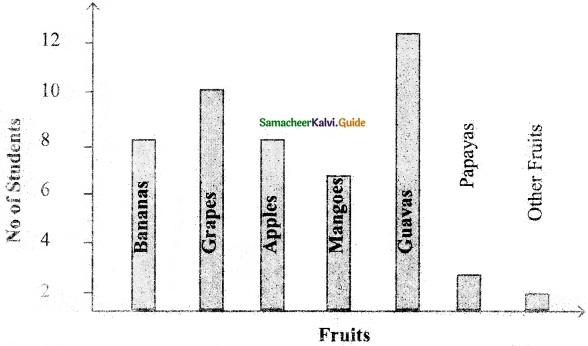Question 4.
The pictograph below gives the number of absentees on different days of the week in class six. Draw the Bar graph for the same.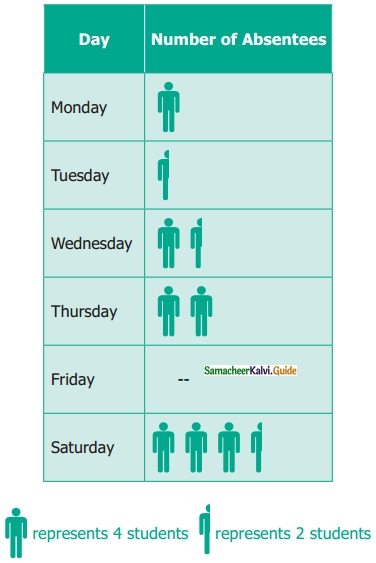Solution:
No of absentees on different days of the week in a class
Scale 1 unit = 2 students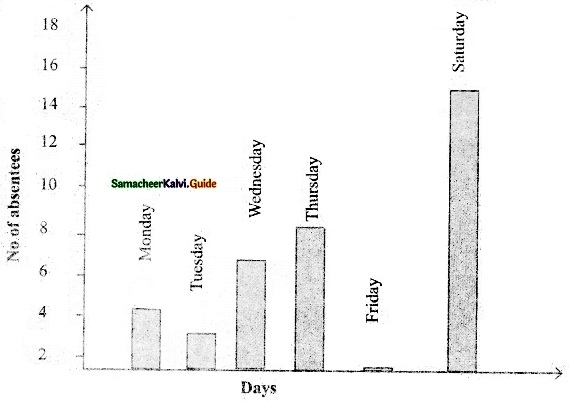Objective Type Questions

Question 5.
A bar graph can be drawn using
(a) Horizontal bars only
(b) Vertical bars only
(c) Both horizontal bars and Vertical bars
(d) Either horizontal bars or vertical bars
Solution:
(d) Either horizontal bars or vertical barsQuestion 6.
The spaces between any two bars in a bar graph
(a) can be different
(b) are the same
(c) are not the same
(d) all of these
Solution:
(b) are the same